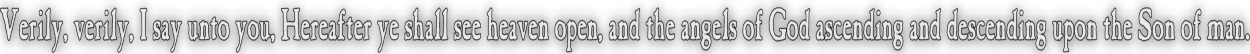None:
Polyps:
Strongs:CreationismLeft, Right, Somewhere In The Middle With (0,a,b,c) a static subgroup and d = 1 with {e,f,g} a static bow, we may have (d,a,b,e,c,g,f) a suitable multiplication. Then we have the natural associations of; (a,b,c) = 1 = d = {e,f,g} (b,e,g) = a = {c,d,f} (c,e,f) = b = {a,d,g} (c,d,g) = e = {a,b,f} (a,f,g) = c = {b,d,e} (b,d,f) = g = {a,c,e} (a,d,e) = f = {b,c,g} Yet if we have our operation on the earthy elements {d,e,f,g} then it follows that the elements {e,f,g} correspond to the subgroups (c,d,g), (a,d,e) and (b,d,f) respectively. (Those groups with intersection unity (d)) So if e = (f ∪ g)c when d=>{} we would also expect {c,d,g} = ({a,d,e} ∪ {b,d,f})c = {a,b,d,e,f}c = {c,g} (Note d={}) Which certainly appears valid, as long as unity is mapped to {} or is "dead". No wonder satan took the opportunity to slay the lamb of God, (unity is as the lamb slain before the throne in revelation). So then the operation of the dialectic as similar to K4 also acts across the right as well as left hands equivalently. (As the hail and fire mingled with blood.) Can we make anything of g*g from before? In the elements {d,e,f,g} with d=>{} we would have g = {d,e,f} yet we would require a different octal for this left hand under the multiplication of say, (g,a,b,f,c,d,e). Then g = (a,b,c) = {d,e,f} (now as with a different choice of unity, "g") and clearly now g*g = {}*{} = (0,a,b,c,d,e,f,g) i.e. the octal as identity. God, we note, is not moved from the static subgroup (a,b,c). We would assert now with confidence that to compare triples in the earthy elements with their "fixed singletons" (a choice from four possible unities) we need to consider those singletons as unity and although in each case there is a similarity to K4, there is only the sense that a singleton not sent to {} is the product of the other two. There is no certain sense of addition modulo 2. Yet if we must press the requirement of a product of g*g, and also d*d, e*e and f*f as equal to the zero element or octal we must be able to "choose" a suitable left hand octal from the four that are possible from the eight C7 groups over the right hand. We assumed that we could shift from one to the other and the revelation states this possibility in the first four trumpets. So, rather than attempt to justify this on the basis of d=1 sent to {}, and e*f=g, e*g=f etc, We should relate the similar relation upon the octal groups that are chosen from the wider "sea", the same required to provide those static triples, those "bows". Once again we have upon our choices of multiplication given a starting "sun" octal with say (a,b,c) static then for any product d*e say, the result may be one of 'f' or 'g' that remain.. We saw this before as the dialectic "Not". Then for d = 1 and e*f = g etc, there are always two octals with (a,b,c) static. Yet for g*g = {}c = (0,a,b,c,d,e,f,g), There are also two octals, but we require g = 1. Within the same octal we now have e*f = d etc, a clear change of operations. Now that we may perform products of e*f = g when d=>{} we only do so when we are using bows, so e*f = {{f,g} ∩ {e,g}}= g These bows are taken from different left handed octals using the same right hand; Then we have a choice of two sets of octals, one in which e*f = d and the other in which e*f = g. One choice will render g = 1 =>{} and the other d = 1 =>{}. (Not counting those where 'e' or 'f' is equal to 1.) So, if our singletons each correspond to a different octal, then d*e = f is a product of two octals onto a third whilst the fourth is "as wormwood" or "death" and is mapped to {}. Then preserving the right hand octal, the prducts of "e*f" etc are actually the products of two octals, one in which e = 1 and another where f = 1. It makes sense then that g*g = {} * {} = (0,a,b,c,d,e,f,g) since the product g*g occurs in only the one octal where g=1 and g is sent to {} by "construction" or "architecture" rather than by any other reason. Continue To Next Page Return To Section Start Return To Previous Page'